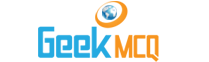CSS :: Scale Of Production and Laws of Returns

1.  Negative returns in the short run imply that:
 A. Average product is negative B. Total product is negative C. Marginal is negative D. Total cost is falling

2.  Economies of scale:
 A. Occur when an increase in input causes a less than proportionate increase in output B. Suggest that firm's marginal cost curve lies above its average cost curve C. Suggest that the firm's marginal cost curve lies above its average cost curve D. None of these

3.  In law of diminishing returns at least one factor:
 A. Must be constant B. Must be owned by the firm C. Must be purchasable D. Must be efficient

4.  Economies of scale are of two kinds:
 A. Temporary and permanent B. Internal and external C. Managerial and industrial D. Natural and artificial

5.  Laws of return apply to firms working in:
 A. Perfect competition B. Monopoly C. Small firm D. All kinds of market situations

6.  During short period, diminishing returns may follow because:
 A. Quantity of labour is fixed B. Quantity is fixed C. Quantity of capital is fixed D. Quantity of any one factor is fixed

7.  When a firm using a fixed amount of land and capital takes on more workers, it finds that marginal product (MP) of labour falls but the average product (AP) of labour rises. This can be explained by the factors that:
 A. The MP of labour is greater than AP of labour B. The additional workers are more efficient C. MP and AP always moves in opposite directions D. None of these

8.  Which of the following is example of external economies of scale?
 A. Discount on purchases of raw materials B. Technical progress leads to development of machines at low price C. Hiring of specialized staff due to increase in scale of production D. A firm starts producing by products

9.  The maximum point on TP curve is at quantity of labour where:
 A. Average physical product of labour is equal to 1 B. MPP of labour is at its maximum C. Curves of APP and MPP of labour intersect D. MPP of labour is zero

10.  When a firm using a fixed amount of land and capital takes on more workers, it finds that marginal product (MP) of labour falls but the average product (AP) of labour rises. This can be explained by the factors that:
 A. The MP of labour is greater than AP of labour B. The additional workers are more efficient C. MP and AP always moves in opposite directions D. (b) and (c)